×
Get Full Access to Introductory Chemistry - 5 Edition - Chapter 6 - Problem 66p
Get Full Access to Introductory Chemistry - 5 Edition - Chapter 6 - Problem 66p

×

# Solved: For each set of molecular models, write a relationship between moles of oxygenISBN: 9780321910295 34

## Solution for problem 66P Chapter 6

Introductory Chemistry | 5th Edition

• Textbook Solutions
• 2901 Step-by-step solutions solved by professors and subject experts
• Get 24/7 help from StudySoup virtual teaching assistantsIntroductory Chemistry | 5th Edition

4 5 1 410 Reviews
19
0
Problem 66P

Problem 66P

For each set of molecular models, write a relationship between moles of oxygen and moles of molecules. Then determine the total number of oxygen atoms present. (H— white; O—red; C—black; S—yellow)

(a)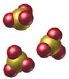(b)(c)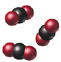Step-by-Step Solution:

Problem 66P

For each set of molecular models, write a relationship between moles of oxygen and moles of molecules. Then determine the total number of oxygen atoms present. (H— white; O—red; C—black; S—yellow)

(a)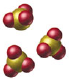(b)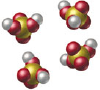(c)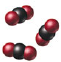Step by step solution

Step 1 of 3

a)

In the given image the compound is -One mole ofcontains three moles of “O” atoms.

Let’s calculate the number of moles of oxygen present in the three moles ofTherefore, 9 oxygen atoms present in the given sample.

___________________________________________________________________________

Step 2 of 3

Step 3 of 3

##### ISBN: 9780321910295

Unlock Textbook Solution